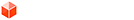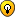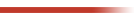## [Code] position of texture pins

### [Code] position of texture pins

Hi,
I searched in the forum everywhere but I'm not sure if there exists already some code for this. Basically I want to find the (possible) position of texture pins - or better - the corners of a texture image on a face.
uvq = uv_helper.get_front_UVQ(vert.position) gives me the texture coordinate when I input a certain model coordinate. What I want is the opposite, ie. to get the model coordinate of the uvq [0,0,1], [0,1,1] etc. At the moment it is like digging in the dark.
I would start my code by "testing" four model coordinates that are arranged as a square on the face and do some (more or less complex) math to find where uvq is [0,0,#] etc.
0
Last edited by Aerilius on Sun Nov 06, 2011 10:14 pm, edited 1 time in total.

Aerilius
PluginStore Author### Re: position of texture pins

Get the UV of two points. From that you can calculate where the origin is. You use the two points to act as two point on a line and then see where that line intersects with the X and Y of the UV coords.

...did that make sense at all?
0
Thomas Thomassen — SketchUp Monkey & Coding addictthomthom
PluginStore Author### Re: position of texture pins

Sounds easier than my approach, but I'm not sure how it would work with non-rectangular distorted textures.

I've got some progress.
I convert 3 world coordinate points into uvq coordinates (API method) and use them as fix points for a barycentric coordinate system. Then I express the texture's corners (uvq [0,0,0] etc.) in barycentric coordinates. With the world coordinates of the 3 fix points I can convert the barycentric texture corners into the world coordinate system.

Unfortunately it works only for affine texture transformations (any scale, rotation, shearing), but no distortion with four texture coordinates. Must be due to the barycentric system, I'll have to search if it can be used also with four fix points.
0

Aerilius
PluginStore Author### [code] position of texture pins

get_texture_pins(face)
Code: Select all
`def get_texture_pins(face)Sketchup.active_model.start_operation("UV test")  tw = Sketchup.create_texture_writer  uv_helper = face.get_UVHelper true, true, tw  # three perpendicular points  v1 = face.vertices.position  v2 = face.vertices.position  vec1 = v1.vector_to(v2)  vec2 = vec1 * face.normal  v3 = v1 - vec2  # their uvq  uv1 = uv_helper.get_front_UVQ(v1)  uv2 = uv_helper.get_front_UVQ(v2)  uv3 = uv_helper.get_front_UVQ(v3)  uv1.to_a.collect!{|x| x /= uv1}  uv2.to_a.collect!{|x| x /= uv2}  uv3.to_a.collect!{|x| x /= uv3}  # texture corners in texture coordinates  t1 = to_barycentric(uv1, uv2, uv3, [0,0,1])  t2 = to_barycentric(uv1, uv2, uv3, [0,1,1])  t3 = to_barycentric(uv1, uv2, uv3, [1,1,1])  t4 = to_barycentric(uv1, uv2, uv3, [1,0,1])  # texture corners in world coordinates  p1 = from_barycentric(v1, v2, v3, t1)  p2 = from_barycentric(v1, v2, v3, t2)  p3 = from_barycentric(v1, v2, v3, t3)  p4 = from_barycentric(v1, v2, v3, t4)  Sketchup.active_model.entities.add_cpoint(p1)  Sketchup.active_model.entities.add_cpoint(p2)  Sketchup.active_model.entities.add_cpoint(p3)  Sketchup.active_model.entities.add_cpoint(p4)Sketchup.active_model.commit_operationend #def get_texture_pinsdef to_barycentric(f1, f2, f3, p)  area = heron(f1, f2, f3).to_f  n = (f2.vector_to(f1))*(f2.vector_to(f3))  # we need to change the algebraic sign when p lays outside of the triangle f1f2f3  area1 = heron(f2, f3, p) * orientation([f2, f3, p], n)  area2 = heron(f3, f1, p) * orientation([f3, f1, p], n)  area3 = heron(f1, f2, p) * orientation([f1, f2, p], n)  b = Geom::Point3d.new()  b.x = area1/area  b.y = area2/area  b.z = area3/area  return bend #def to_barycentricdef from_barycentric(f1, f2, f3, b)  p = Geom::Point3d.new()  p.x = f1.x*b.x + f2.x*b.y + f3.x*b.z  p.y = f1.y*b.x + f2.y*b.y + f3.y*b.z  p.z = f1.z*b.x + f2.z*b.y + f3.z*b.z  return pend #def from_barycentric# Heron's formular, area of a triangledef heron(p1, p2, p3)  a = p1.distance(p2)  b = p2.distance(p3)  c = p3.distance(p1)  s = 0.5*(a+b+c) # semiperimeter  area = Math.sqrt( (s*(s-a)*(s-b)*(s-c)).abs )  return areaend #def heron# points are oriented counter-clockwise (1) or clockwise in comparison to normal1def orientation(points, normal1)  orientation = 1  normal2 = (points.vector_to(points))*(points.vector_to(points))  orientation = -1 if normal2!=[0,0,0] && !normal1.samedirection?(normal2)  return orientationend #def orientation`
0

Aerilius
PluginStore Author### [Code] position of texture pins

Aerilius wrote:Unfortunately it works only for affine texture transformations

As said, my own strategy only dealt well with affine transformations. I did some research and found the proper code below for affine/projective coordinate transformations with matrix calculation etc. Translated from C code to Ruby.

Usage: get_texture_pins(face, side)
- with side = true for frontface, false for backface
- returns: Array of 4 Geom::Point3d
Note: SketchUp dynamically places the texture pins where the user clicks, thus they can be translated about u/v +/-1

May it be useful.
Code: Select all
`### from:# Paul S. Heckbert, Fundamentals of Texture Mapping and Image Warping, 1989# pages 68-73### determinant of a 2x2 matrix#def det2(a, b, c, d)  return a*d - b*cend #def det2### matrix multiply: c = a*b#def mx3_mul(a, b)  c = [[],[],[]]  c = a*b + a*b + a*b  c = a*b + a*b + a*b  c = a*b + a*b + a*b  c = a*b + a*b + a*b  c = a*b + a*b + a*b  c = a*b + a*b + a*b  c = a*b + a*b + a*b  c = a*b + a*b + a*b  c = a*b + a*b + a*b  return cend #def mx3_mul### transform point p by matrix a: q = p*a#def mx3d_transform(p, a)  q = []  q = p*a + p*a + p*a  q = p*a + p*a + p*a  q = p*a + p*a + p*a  return qend #def mx3d_transform### invert matrix#def mx_invert(a)  b = [[],[],[]]  b = a*a - a*a  b = a*a - a*a  b = a*a - a*a  b = a*a - a*a  b = a*a - a*a  b = a*a - a*a  b = a*a - a*a  b = a*a - a*a  b = a*a - a*a  return bend #dev mx_invert### pmap_square_quad:# find the mapping matrix for transforming a # unit square [0,0], [1,0], [1,1], [0,1]# into a quad#def pmap_square_quad(face, front=true)  tolerance = 0.000001  tw = Sketchup.create_texture_writer  uv_helper = face.get_UVHelper true, true, tw  # four points of a square  v0 = face.vertices.position  v1 = face.vertices.position  vec0 = v0.vector_to(v1)#.normalize ?  v1 = v0 + vec0  vec1 = (vec0 * face.normal)#.normalize ?  v2 = v0 + vec0 + vec1  v3 = v0 + vec1  # their uvq  if front==true    uv0 = uv_helper.get_front_UVQ(v0)    uv1 = uv_helper.get_front_UVQ(v1)    uv2 = uv_helper.get_front_UVQ(v2)    uv3 = uv_helper.get_front_UVQ(v3)  else    uv0 = uv_helper.get_back_UVQ(v0)    uv1 = uv_helper.get_back_UVQ(v1)    uv2 = uv_helper.get_back_UVQ(v2)    uv3 = uv_helper.get_back_UVQ(v3)  end  uv0 = uv0.to_a.collect!{|x| x /= uv0}  uv1 = uv1.to_a.collect!{|x| x /= uv1}  uv2 = uv2.to_a.collect!{|x| x /= uv2}  uv3 = uv3.to_a.collect!{|x| x /= uv3}  # transformation from square to quad  t=[[],[],[]]  px = uv0.x - uv1.x + uv2.x - uv3.x  py = uv0.y - uv1.y + uv2.y - uv3.y   # affine  if px.abs<tolerance && py.abs<tolerance # zero or near zero  puts "affine"    t = uv1.x - uv0.x    t = uv2.x - uv1.x    t = uv0.x    t = uv1.y - uv0.y    t = uv2.y - uv1.y    t = uv0.y    t = 0    t = 0    t = 1    return t   # projective  else  puts "projective"    dx1 = uv1.x - uv2.x    dx2 = uv3.x - uv2.x    dy1 = uv1.y - uv2.y    dy2 = uv3.y - uv2.y    del = det2(dx1, dx2, dy1, dy2)    return nil if del==0    t = det2(px, dx2, py, dy2)/del    t = det2(dx1, px, dy1, py)/del    t = 1.0    t = uv1.x - uv0.x + t * uv1.x    t = uv3.x - uv0.x + t * uv3.x    t = uv0.x    t = uv1.y - uv0.y + t * uv1.y    t = uv3.y - uv0.y + t * uv3.y    t = uv0.y    return t  endend### convert a specific uvq into xyz of object coordinate system# opposite of UVhelper.get_front_UVQ([x,y,z])#def UVQ_to_XYZ(uvq, face, transformation)  # origin point and two vectors in 3d coordinates  v0 = face.vertices.position  v1 = face.vertices.position  vec0 = v0.vector_to(v1)#.normalize ?  vec1 = (vec0 * face.normal)#.normalize ?  # texture coordinates to 2d coordinates  xyw = mx3d_transform(uvq, mx_invert(transformation))  xyw.x /= xyw  xyw.y /= xyw  # 2d coordinates to 3d coordinates  xyz = Geom::Point3d.new()  xyz.x = v0.x + vec0.x * xyw.x + vec1.x * xyw.y  xyz.y = v0.y + vec0.y * xyw.x + vec1.y * xyw.y  xyz.z = v0.z + vec0.z * xyw.x + vec1.z * xyw.y  return xyzend #def UVQ_to_XYZ### get the object coordinates of the texture pins of a face (front, or back)## Note that SketchUp places the texture pins near where the user right clicks # to position the texture. This means it could also be translated:#  [m+0,n+0,1]#  [m+1,n+0,1]#  [m+1,n+1,1]#  [m+0,n+1,1]def get_texture_pins(face, front=true)  Sketchup.active_model.start_operation "get texture pins"  t = pmap_square_quad(face, front)  pins = [ UVQ_to_XYZ([0,0,1], face, t),           UVQ_to_XYZ([1,0,1], face, t),           UVQ_to_XYZ([1,1,1], face, t),           UVQ_to_XYZ([0,1,1], face, t)         ]  # example: show pins with construction points  pins.each{|p| Sketchup.active_model.entities.add_cpoint(p)}  Sketchup.active_model.commit_operation  return pinsend #def get_texture_pins`
0

Aerilius
PluginStore Author### Re: [Code] position of texture pins

That's very interesting.

btw...
Aerilius wrote:Note: SketchUp dynamically places the texture pins where the user clicks, thus they can be translated about u/v +/-1

From what I see, that is only true if the texture has not been positioned. If the use has positioned the texture, then the pins appear where they where last placed.
0
Thomas Thomassen — SketchUp Monkey & Coding addictthomthom
PluginStore Author### Re: [Code] position of texture pins

Btw, where was that C code?

Could be interesting to have that in a C Extension...
0
Thomas Thomassen — SketchUp Monkey & Coding addictthomthom
PluginStore Author### Re: [Code] position of texture pins

Btw, where was that C code?

It's available as a pdf at the bottom of that page: http://www.cs.cmu.edu/~ph/

thomthom wrote:If the use has positioned the texture, then the pins appear where they where last placed.
I tested it again. It's true, when the user has changed the pins from their default positioning, they stick fixed. But still the uvq sometimes don't match the pins (once I got [-1,0,1] for the red pin).
0

Aerilius
PluginStore Author### Re: [Code] position of texture pins

I suddenly got a bit more understanding of what we actually do here...So this whole code is actually much simpler. SketchUp gives us a Q coordinate (that we didn't use so far). In fact we don't need a 4×4 transformation matrix (rectangle ←→ quadrilateral), but a 3×3 matrix is enough. That means we can get rid of very expansive calculations and even use SketchUp's native Geom::Transformation (which is 3×3). All the details in the code below and in this thread:

Code: Select all
`module AE;end # (replace this by your author/company namespace!)class AE::UVHelper# This initializes our UVHelper. It calculates the transformation matrix between# model space (XYZ) and texture space (UVQ). We also create an instance of SketchUp::UVHelper# for faster conversion from model to texture space (but it can't do the reverse).## @param [Sketchup::Face] face The face on which we want to convert coordinates.# @param [Sketchup::Face] on_front True if you want the texture coordinates for the front face, false if not. Defaults to true.# @param [Sketchup::Face] on_back True if you want the texture coordinates for the back face, false if not. Defaults to true.# @param [Sketchup::TextureWriter] tw A TextureWriter object.## @return [AE::UVHelper]def initialize(face, on_front=true, on_back=true, tw=Sketchup.create_texture_writer)  @face = face  ### Get the native UVHelper for for conversion from XYZ to UVQ.  @uvh = face.get_UVHelper(on_front, on_back)  ### Get a transformation matrix for UVQ to XYZ.  # Since every quadrilateral can be understood as a perspectivic projection of a 3d rectangle,  # we do not need to find a transformation [square <-> quadrilateral] (4x4 matrix) but only an  # affine transformation in 3d space (3x3 matrix, can use Geom::Transformation!).  #  # Get 3 points of a square of size 1*1. It spans a local 2d space of the face's plane (XY).  p0 = face.vertices.position  p1 = face.vertices.position  vec01 = p0.vector_to(p1).normalize  p1 = p0 + vec01  vec12 = (vec01 * face.normal)  p2 = p1 + vec12  # For one side of the face  get_transformation = Proc.new{|side|    # Get their uvq    uvq = [p0,p1,p2].collect{|p|      get_UVQ(p, side)    }    # Get the transformation of the between model space (XYZ) and the face (XY).    txyz = Geom::Transformation.axes(p0, vec01, vec12, face.normal) # * scaling(1)    # Get the transformation of the between the face (XY) and UVQ.    # Since we have input a square, it corresponds to a rectangle in UVQ space    # and we may use an axes transformation (that's why 3 points are enough).    vecuvq01 = uvq.vector_to(uvq)    vecuvq12 = uvq.vector_to(uvq)    vecuvqn = vecuvq01*vecuvq12    tuvq = Geom::Transformation.axes(uvq, vecuvq01, vecuvq12, vecuvq01*vecuvq12) *           Geom::Transformation.scaling(ORIGIN, vecuvq01.length, vecuvq12.length, 1)    # The combined transformation from texture space to model space.    t = txyz * tuvq.inverse    # The projection plane (necessary if confronted with UV and Q=1)    plane = [uvq, vecuvqn]    [t, plane]  }  @t_front_uvq2xyz = @t_back_uvq2xyz = Geom::Transformation.new()  @plane_front = @plane_back = [ORIGIN, Z_AXIS]  @t_front_uvq2xyz, @plane_front = get_transformation.call(true) if on_front  @t_back_uvq2xyz, @plane_back = get_transformation.call(false) if on_backend# Wrapper method for Sketchup::UVHelper (more useful with front/back Boolean)## @param [Geom::Point3d, Array] xyz A point in model space.# @param [Boolean] on_front Whether you want the texture coordinates for the front face or the back face.## @return [Geom::Point3d] Point The corresponding point in texture space (UVQ).def get_UVQ(xyz, on_front=true)  return (on_front)? @uvh.get_front_UVQ(xyz) : @uvh.get_back_UVQ(xyz)end# Get the coordinates in model space from given coordinates in texture space.## @param [Geom::Point3d, Array] uvq A point in texture space (UV or UVQ).# @param [Boolean] on_front Whether you want the model coordinates for the front face or the back face.## @return [Geom::Point3d] Point The corresponding point in model space (XYZ).def get_XYZ(uvq, on_front=true)  # If the input is a 2d texture coordinate (UV with Q==1) it may not lay on the   # projection plane and our transformation won't work with a 3x3 matrix.   # Thus find the correct Q value on the projection plane.  vec_q = [uvq*2, uvq*2, (uvq||1)*2]  uvq = Geom.intersect_line_plane([uvq, vec_q], (on_front)? @plane_front : @plane_back)  return uvq.transform((on_front)? @t_front_uvq2xyz : @t_back_uvq2xyz)end# Get the coordinates in model space from given coordinates in texture space.## @param [Geom::Point3d, Array] Point A point in texture space (UV or UVQ).# @param [Boolean] on_front Whether you want the texture pins for the front face or the back face.## @return [Geom::Point3d] Point The corresponding point in model space (XYZ).def get_texture_pins(on_front=true)  pins = [ get_XYZ([0,0,1], on_front),           get_XYZ([1,0,1], on_front),           get_XYZ([1,1,1], on_front),           get_XYZ([0,1,1], on_front),         ]  # example: show pins with construction points  Sketchup.active_model.start_operation "get texture pins"  pins.each{|p| Sketchup.active_model.entities.add_cpoint(p)}  Sketchup.active_model.commit_operation  return pinsend #def get_texture_pinsend`
0
Last edited by Aerilius on Tue Aug 14, 2012 4:22 pm, edited 1 time in total.

Aerilius
PluginStore Author### Re: [Code] position of texture pins

You sure you don't need the fourth in order to get the skew and distortion?
0
Thomas Thomassen — SketchUp Monkey & Coding addictthomthom
PluginStore Author### Re: [Code] position of texture pins

UV.png

get_pins.png
0
Thomas Thomassen — SketchUp Monkey & Coding addictthomthom
PluginStore Author### Re: [Code] position of texture pins

Hmm, about skewing I'm not sure, but I see it works without. I think for 4 points there are 8 degrees of freedom (? 4*[U,V] if Q=1 is constant). With varying Q it works also with 3 points 3*[U,V,Q]=9 (?).

Edit: I haven't experienced before what your image/skp shows. One construction point is across the inversion horizont of the texture (where it grows after shrinking). I have to see what can be done...
0

Aerilius
PluginStore Author### Re: [Code] position of texture pins

When you position a texture SketchUp let you provide the following Point3d and UV pairs:

1x : Position
2x : Position and Scale Uniform
3x : Position and Skew
4x : Perspective Distortion

So for the reverse one does need all four points as well - without it you lose the data.

Many render engines struggle with SketchUp's distorted textures - mainly because they read the UV data from the vertices of the face. That fails when a triangle has a distorted texture applies. And it is why SU's UV mapping methods accepts 3D points that are on the plane of the face instead of using the vertices.

Several render engines works around it by triangulating the mesh and reading the texture for each triangle - it doesn't give correct UV map though - as they only read 3 point they only get a skewed map. For some geometry you hardly notice it, but other times it is very visible.
0
Thomas Thomassen — SketchUp Monkey & Coding addictthomthom
PluginStore Author### Re: [Code] position of texture pins

But since a texture is 2d, it has 8 degrees of freedom on the plane. You need four points with 2d coordinates (UV) to sufficiently define texture position/skew/distortion. With 3d texture coordinates, we have already enough data in three 3d points (UVQ) (the third Q coordinate contains the missing info).
0

Aerilius
PluginStore Author### Re: [Code] position of texture pins

Aerilius wrote:With 3d texture coordinates, we have already enough data in three 3d points (UVQ) (the third Q coordinate contains the missing info).

I never worked out how to use that value... I tried asking the SketchUppers - but there feedback hasn't really provided anything...
0
Thomas Thomassen — SketchUp Monkey & Coding addictthomthom
PluginStore Author### Re: [Code] position of texture pins

I am so frustrated that I don’t possess the knowledge yet to understand most of this discussion. Correct me if I am wrong but could this code make animating textures possible? If so I am very interested in trying to figure how I can do this myself.
0renderiza